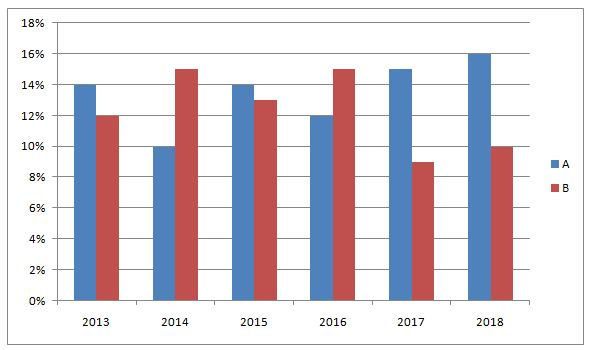# gkrecall

We brings you daily Current affairs, daily Current Affairs Quiz, weekly Current Affairs, weekly Current Affairs Quiz to enhance your preparation for upcoming exams. We also provide articles related to quant, reasoning, English, ssc etc .

## QUANT PRACTICE SET 2 FOR RRB PO & CLERK PRELIM 2020

RRB Clerk and RRB PO Prelims exam are going to be held in the upcoming months. We have already provided you with the PDFs of many topics of Quantitative Aptitude like Simplification/Approximation, Number Series,datainterpretation.important Arithmetic questions to prepare for RRB Clerk and PO Pre exam. Practicing these questions will help you to know about the level of the questions. To increase your speed and accuracy, enhance your calculations.

Web

### START QUIZ

Directions (1 – 5): What Approximate value should come in place of question mark (?) in the following questions?
1) 820.01 ÷ 21 × 2.99 + ? = 240
a) 105
b) 173
c) 123
d) 234
e) 143
2) 299 ÷ 12 × 13.95 + ? = (24.02)2
a) 285
b) 225
c) 325
d) 150
e) 185
3) 92 × 576 ÷ √1296 = (?)3 + √49
a) 3
b) 14
c) 11
d) 27
e) None of these
4) (21.5% of 999)(1/3) + (43% of 601)1/2 = ?
a) 18
b) 22
c) 26
d) 30
e) None of these
5)  40.005% of 439.998 +? % of 655.011 = 228.5
a) 8
b) 17
c) 12
d) 20
e) 5
Directions (Q. 6 – 10): Study the following information carefully and answer the given questions.
The following bar graph shows the profit % earned by two different companies in 6 different years.6) If the expenditure of company A in the year 2013 is Rs. 138 lakhs, which is 25 % less than the income of company B in the year 2014, then find the sum of the income of company A in the year 2013 and theexpenditure of company B in the year 2014?
a) 317.32 lakhs
b) 378.46 lakhs
c) 405.74 lakhs
d) 456.18 lakhs
e) None of these
7) If the income of company A in the year 2015 and the income of company B in the year 2017 is same, find the ratio between the expenditure of company A in the year 2015 to that of the expenditure of company B in the year 2017?
a) 23 : 27
b) 57 : 62
c) 177 : 196
d) 109 : 114
e) None of these
8) If the income of company A in the year 2015 is 95 lakhs and the expenditure of company B in the year 2016 is 110 lakhs, the expenditure of company A in the year 2015 is approximately what percentage more/less than the income of company B in the year 2016?
a) 26 % more
b) 30 % less
c) 26 % less
d) 30 % more
e) 35 % less
9) If the expenditure of company A and B in the year 2018 is 125 lakhs and 140 lakhs respectively, then find the average income of company A and B in the year 2018?
a) 149.5 lakhs
b) 153.5 lakhs
c) 146 lakhs
d) 158 lakhs
e) None of these
10) If the expenditure of company A in the year 2017 is 120 lakhs and the income of company B in the year 2018 is 154 lakhs, then find the difference between the income of company A in the year 2017 to that of the expenditure of company B in the year 2018?
a) 5 lakhs
b) 6 lakhs
c) 2 lakhs
d) 4 lakhs
e) None of these

### SOLUTIONS:

Direction (1-5) :
820 ÷ 21 × 3 +? ≈ 240
Or, 39 × 3 +? ≈ 240
Or,? ≈ 240 – 117 = 123
? ≈ (24.02)2 – 299 ÷ 12 × 13.95
≈ 576 – 25 × 14 = 576 – 350 = 226 ≈ 225
(?)3 + √49 = 92 × 876 ÷ √1296
(?)3 + 7 = 92 × 576 ÷ 36
(?)3 + 7 = 92 × 576 ÷36
(?)3 = 1465
?= 11.3
? = (21.5 * 10)1/3 + (43 * 6)1/2
? = (215)(1/3) + (258)(1/2)
? = (63)(1/3) + (162)(1/2)
? = 6 + 16 = 22
40 * (440/100) + ?/100 * 655 = 228
4*44 + ? * 6.55 = 229
? = (229 – 176) / 7
? = 53/7 = 7.5 = 8
Directions (6-10):
The expenditure of company A in the year 2013 = Rs. 138 lakhs
The income of company A in the year 2013
= > 138*(114/100) = 157.32 lakhs
The income of company B in the year 2014 = 138*(100/75) = 184 lakhs
The expenditure of company B in the year 2014
= >184*(100/115) = 160 lakhs
Required sum = 157.32 + 160 = 317.32 lakhs
The income of company A in the year 2015 = The income of company B in the year 2017 = x
The expenditure of company A in the year 2015 = x*(100/114)
The expenditure of company B in the year 2017 = x*(100/109)
Required ratio = [x*(100/114)] : [x*(100/109)]
= >109 : 114
The income of company A in the year 2015 = 95 lakhs
The expenditure of company A in the year 2015
= >95* (100/114) = 83.33 lakhs
The expenditure of company B in the year 2016 = 110 lakhs
The income of company B in the year 2016
= > 110*(115/100) = 126.5 lakhs
Required % = [(126.5 – 88.33)/126.5]*100 = 30.17 % = 30 % less